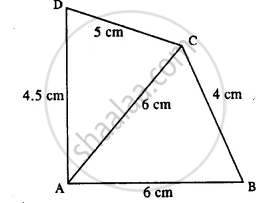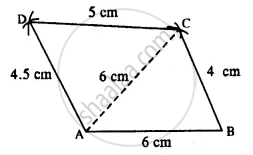# Construct a Quadrilateral Abcd; If: Ab = 6 Cm = Ac, Bc = 4 Cm, Cd = 5 Cm and Ad = 4.5 Cm. - Mathematics

Sum

AB = 6 cm = AC, BC = 4 cm, CD = 5 cm and AD = 4.5 cm.

#### Solution

The rough figure is as shown below.The actual quadrilateral is constructed as follows with the help of the above rough figure.Steps :

1. Draw AB = 6 cm.
2. From A and B, draw arcs of radii 6 cm and 4 cm which cut at C.
3. From A and C, draw arcs of radii 4.5 cm and 5 cm respectively which intersect at D.
4. Join BC, CD, and DA. Thus ABCD is the required quadrilateral.
Is there an error in this question or solution?

#### APPEARS IN

Selina Concise Mathematics Class 8 ICSE
Chapter 18 Constructions
Exercise 18 (D) | Q 1.5 | Page 211